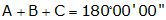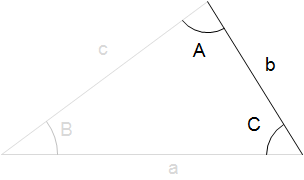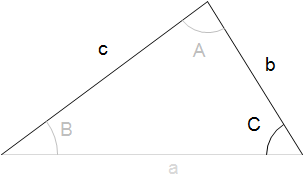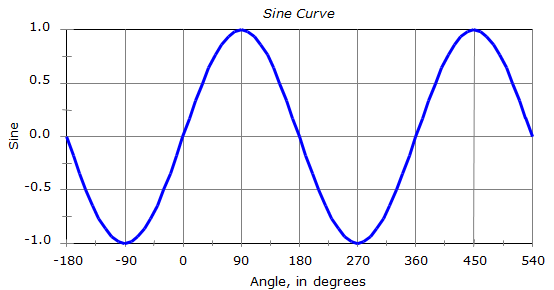## 1. Oblique triangles

### a. Formulae

An oblique triangle is one in which individual angles are greater than 0° and less than 180°. A right triangle is a special oblique triangle in which one angle is exactly 90°.

A plane triangle has six parts: three angles and three sides.Figure B-1 Triangle Parts

Equations for triangle trigonometry are:

 Angle ConditionEquation B-1 Law of SinesEquation B-2 Law of CosinesEquation B-3

A right triangle is a special case where one angle is exactly 90°. If we apply the Law of Cosines, Equation B-3, to a right triangle:The Law of Cosines becomes the Pythagorean Theorem. Figure B-2 Right triangle

### b. Solving triangles

In order to geometrically fix a triangle you must start with at least three parts and one of those must be a side. Why a side? Because fixing the angles alone does not constrain the size of the triangle.These two triangles have identical angles but are different sizes. Figure B-2 Angles Don't Fix Triangle

Starting with three parts of a triangle, there is only one way to begin solving for another of its parts. Once a fourth part is determined, there are more ways to solve the remaing parts.

For example:Given three sides of a triangle, how would you begin solving for other parts of it?Start with the Law of Cosines to solve for one angle. a2=b2+c2-2x(bc)xCos(A) Can then use Law of Cosines or Law of Sines to solve for next angle. Can then use Law of Cosines or Law of Sines to solve for next angle. Last angle can be solved by Law of Cosines, Law of Sines, or Angle ConditionHow about two sides and an included angle?Start with the Law of Cosines to solve for side a (known values in red): a2=b2+c2-2x(bc)xCos(A) Can then use Law of Cosines or Law of Sines to solve for another angle. Last angle can be solved by Law of Cosines, Law of Sines, or Angle Condition

How would you solve these triangles?Two angles and the included side.Two angles an a non-included side.Two sides and a non-included angle.

### c. Area

Another attribute of a triangle is its area which can be determined by one of two equations:

 Using two sides and an included angle.Equation B-4 Using all three sides - Heron's Formula.Equation B-5

Given two parts of a triangle and its area, you should be able to determine its other parts, right?Starting with two sides and an area...Solve angle A using the area equation: Area = 1/2(bc)xCos (A) Then use Law of Cosines to solve side a a2=b2+c2-2(bc)Cos(A) Then.....How about starting with an angle, opposite side, and triangle area?

## 2. Trigonometric Functions

### a. Sine; Law of Sines

The sine of any angle falls between -1.0 and +1.0. Refer to the sine curve plot in Figure B-4 to see how angles and their sines relate. This curve repeats itself every 360°;
so sin(-340°) = sin(20°) = sin(380°)...Figure B-4

Taking the arcsine (a.k.a. inverse sine, sin-1) of a number between -1.0 and +1.0 on a calculator will always return an angle between -90° and +90°.

For example:

Sin(101°) is 0.981627

Sin-1(0.866025) is 79°

We started with 101° and wound up with 79°.

Sly obersverse that we are, we notice that 101°+79° is 180°

Table B-1 show some other angles, their sine behavior, relationship between the beginnng and ending angles.

 Table B-1 α x=sin (α) sin-1(x) relationship 30° +0.50000 +30° α 150° +0.50000 +30° 180°-α 210° -0.50000 -30° 180°-α 330° -0.50000 -30° 360°+α

All of the angles in the third colum are between -90° and +90°. This is shown graphically in Figure B-5.Figure B-5 ArcSine Angle Range

A triangle may have an angle which is greater than 90° but if you are solving that angle using the Law of Sines, the computed angle will be less than 90°. Although no single angle in a triangle may exceed 180° the Law of Sines can result in two angles both less than 180°.

For example sin-1(0.5) can be 30° or 150° - both meet the sine condition but only one meets the triangle condition. A calculator will always return 30° even though the “true” angle is 150°. How do you know which is correct? It depends on the triangle.

#### (1) Example

In triangle ABC, a = 12.4', b = 8.7', and B = 36°40'.

Compute the remaining angles and side.

From the Law of SinesThere are two angles whose sine is +0.85112

A = 58°20' and A = (180°00' - 58°20') = 121°40', Figure B-6.Figure B-6 Multiple Angles with Same Sine

That means there are two different triangle solutions, Figure B-7Figure B-7 Two Possible Angle Values

Consider point C as the center of an arc of radius 8.7'.

This arc can intersect the remaining side at two points; A1 and A2.

Isolating the two triangles and solving their remaining components:

For A = 58°20'Figure B-8 First Solution

Angle conditionLaw of SinesFor A = 121°40'Figure B-9 Second Solution

Angle conditionLaw of Sines#### (2) Example 2

Compute the angle B in the following triangle:Figure B-10 Example 2

The missing angle can be computed two ways:

Law of SinesAngle conditionAs a result there are two "correct" answers fot the missing angle:

sin(108°) = sin(72°) = 0.95105652

Ans since neither exceeds 180°, either can be an angle of a triangle,

but only 108° fits both the Law of Sines and the Angle condition.

 Be careful when using the Law of Sines to solve for an unknown angle – there could be two possible answers only one of which will fit the particular triangle.

### b. Cosine; Law of Cosines

The cosine of any angle falls between -1.0 and +1.0. Refer to the cosine curve plot in Figure B-11 to see how angles and their cosines relate. This curve repeats itself every 360°; so cos(-340°) = cos(20°) = cos(380°)...

The cosine curve is identical to the sine curve except its phase differs by 90°.Figure B-11

Taking the arccos (a.k.a. inverse cosine, cos-1) of a number between +1.0 and -1.0 on a calculator will always return an angle between 0° and 180°; for example:

 Table B-2 α x=sin (α) sin-1(x) 60° +0.50000 +60° 300° +0.50000 +60° 120° -0.50000 +120° -240° -0.50000 +120°

All of the angles in the third colum are between 0° and +180°. This is shown graphically in Figure B-12.Figure B-12 ArcCosine Angle Range

Using the Law of Cosines will not cause an ambiguous solution as does the Law of Sines since any single angle in a triangle cannot exceed 180°.

To solve a triangle using the Law of Cosines you must have either three sides, or, two sides and an angle.

#### (1) Example 1

Compute the value of the angle R in the triangle below:Figure B-13 Example

From the Law of Cosines:The Law of Cosines returns only one legitimate value when solving triangles.

### c. Tangent

Unlike sine and cosine, the tangent of any angle not limited to the range of -1.0 to +1.0. As a matter of fact, the tangent range is ±∞ (infinity). You can see that the tangent function plot is not sinusoidal as are the sine and cosine plots. And unlike the other two it repeats itself every 180°;Figure B-14

The tangent curve is asymptotic at 90°, 270°, 450°, etc. Asymptotic means the curve gets close to, but never reaches, those values.
so tan(90°) = tan(270°) = ... = infinity

Try evaluating tan(90°) on your calculator; you’ll probably get an error statement of sorts. Then try tan(89.99999°); you should get a pretty big number. Why is that?

Recall that tan(α) = sin(α) / cos(α)

At 90°, cos(90°) = 0 so you get division by 0, hence tan(90°) = ∞.

There is a Law of Tangents, but we don't generally use it to solve triangles since the Laws of Sines or Cosines can be used instead. For extra credit, find and memorize it.